Скачать презентацию A man tries to get on

e38005a2041806a7f9f7cfb63ce762cf.ppt

• Количество слайдов: 7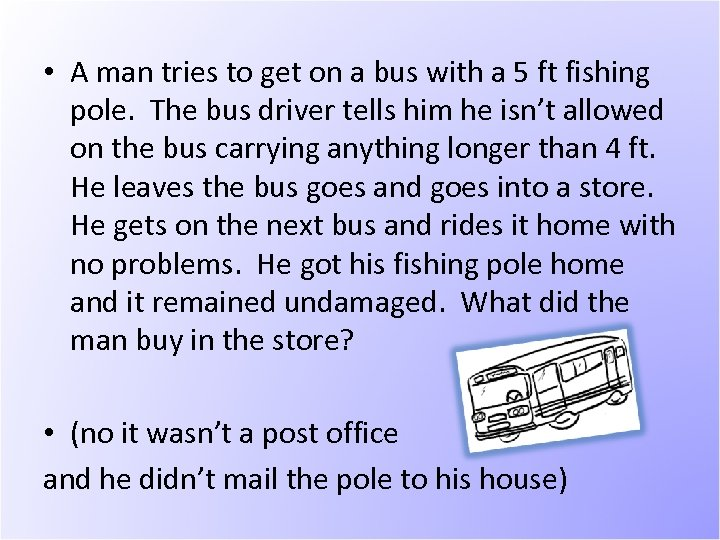• A man tries to get on a bus with a 5 ft fishing pole. The bus driver tells him he isn’t allowed on the bus carrying anything longer than 4 ft. He leaves the bus goes and goes into a store. He gets on the next bus and rides it home with no problems. He got his fishing pole home and it remained undamaged. What did the man buy in the store? • (no it wasn’t a post office and he didn’t mail the pole to his house)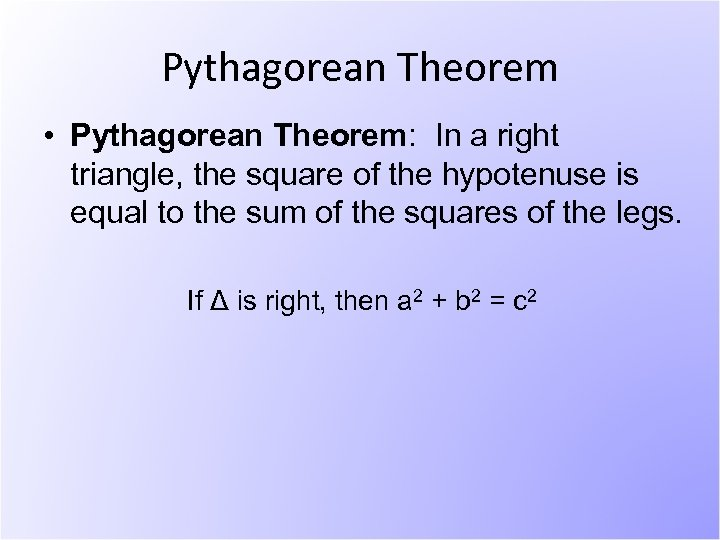Pythagorean Theorem • Pythagorean Theorem: In a right triangle, the square of the hypotenuse is equal to the sum of the squares of the legs. If Δ is right, then a 2 + b 2 = c 2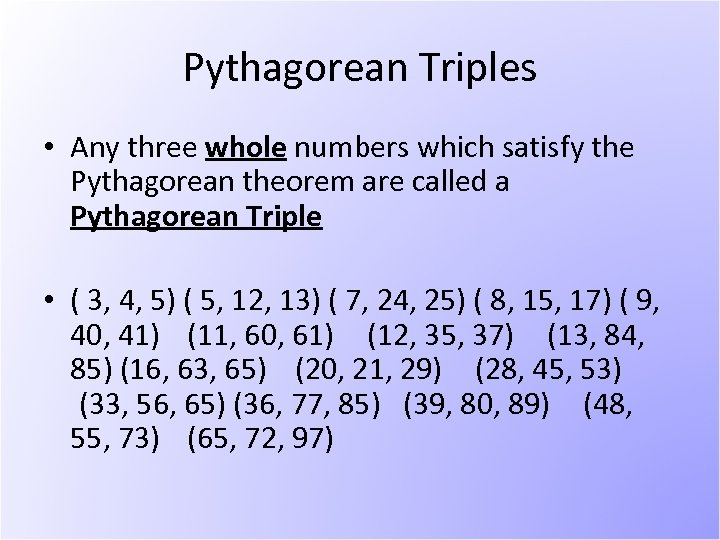Pythagorean Triples • Any three whole numbers which satisfy the Pythagorean theorem are called a Pythagorean Triple • ( 3, 4, 5) ( 5, 12, 13) ( 7, 24, 25) ( 8, 15, 17) ( 9, 40, 41) (11, 60, 61) (12, 35, 37) (13, 84, 85) (16, 63, 65) (20, 21, 29) (28, 45, 53) (33, 56, 65) (36, 77, 85) (39, 80, 89) (48, 55, 73) (65, 72, 97)Proofs • Over 500 unique proofs of the Pythagorean theorem! • http: //www. cut-theknot. org/pythagoras/index. shtml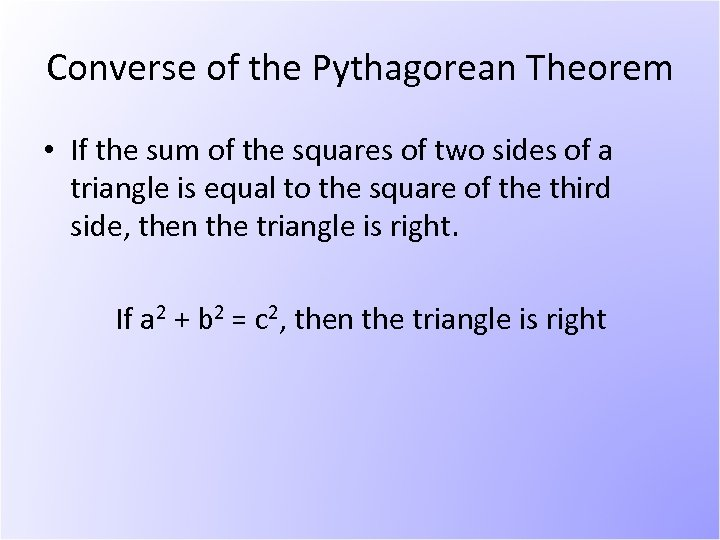Converse of the Pythagorean Theorem • If the sum of the squares of two sides of a triangle is equal to the square of the third side, then the triangle is right. If a 2 + b 2 = c 2, then the triangle is right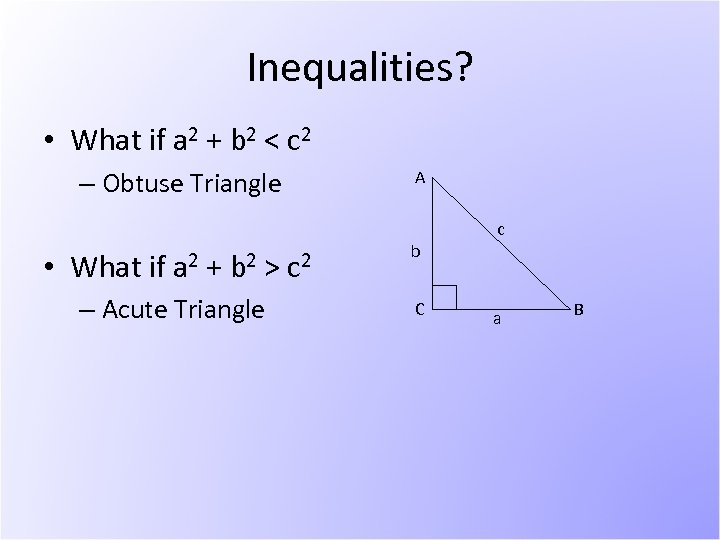Inequalities? • What if a 2 + b 2 < c 2 – Obtuse Triangle • What if a 2 + b 2 > – Acute Triangle A c 2 b C c a B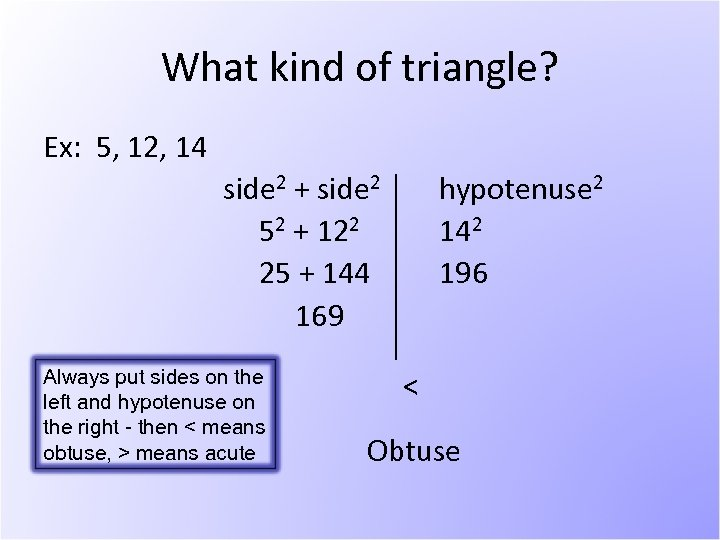What kind of triangle? Ex: 5, 12, 14 side 2 + side 2 52 + 122 25 + 144 169 Always put sides on the left and hypotenuse on the right - then < means obtuse, > means acute hypotenuse 2 142 196 < Obtuse